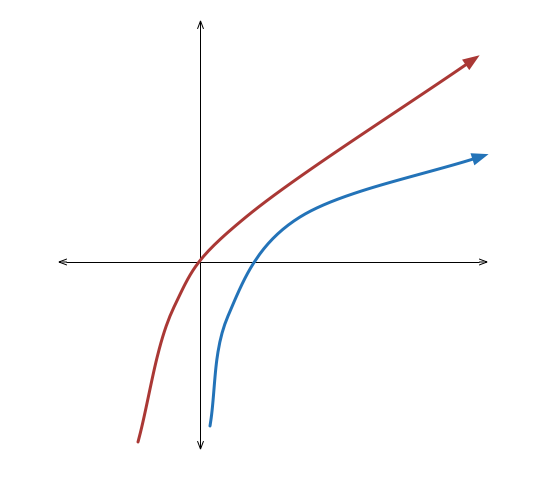# Fun House Mirror

Algebra Level 2Given the graph $y = \ln x$, which of these statements describes the transformations to get the graph of $y = \ln (4x^2 + 4x + 1)$ for $x > - \frac{1}{2}$?

$\quad \text{(1)}$ Translate to the left by 1 and up by $\ln 4$, then scale vertically by 2.

$\quad \text{(2)}$ Translate to the left by $\frac{1}{2}$ and up by $\ln 2$, then scale vertically by 2.

$\quad \text{(3)}$ Translate to the left by 1 and up by $\ln 2$, then scale vertically by 2.

$\quad \text{(4)}$ Translate to the left by $\frac{1}{2}$ and up by $\ln 4$, then scale vertically by 2.

×